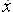Go to content
To differentiate composite functions of the form f(g(x)) we use the chain rule (or "function of a function" rule). The derivative of the function of a function f(g(x)) can be expressed as: f'(g(x)).g'(x)
Alternatively if y=f(u) and u = g(x) then \displaystyle \frac{dy}{dx} = \frac{dy}{du} \times \frac{du}{dx}

## Summary/Background

The chain rule is also known as the "function of a function" rule. It can be stated in a number of ways.
If y = f(u), u = g(x) then \displaystyle\frac{dy}{dx} = \frac{dy}{du}\times \frac{du}{dx}
Here are some more examples:
\displaystyle \frac{d((x+1)^6)}{dx} = 6(x+1)^5.1 = 6(x+1)^5
\displaystyle \frac{d(e^{10x})}{dx} = e^{10x}.10 = 10e^{10x}
\displaystyle \frac{d( \ln x^2)}{dx} = \frac{1}{x^2}.2x = \frac{2}{x}You can get a better display of the maths by downloading special TeX fonts from jsMath. In the meantime, we will do the best we can with the fonts you have, but it may not be pretty and some equations may not be rendered correctly.

## Glossary

### chain rule

A rule for differentiating a function of a function:
dy/dx = dy/du x du/dx.

### composite

made of a combination of simpler shapes or bodies

### derivative

rate of change, dy/dx, f'(x),, Dx.

### differentiate

to find the derivative of a function

### function

A rule that connects one value in one set with one and only one value in another set.

### rule

A method for connecting one value with another.

### union

The union of two sets A and B is the set containing all the elements of A and B.

Full Glossary List

## This question appears in the following syllabi:

SyllabusModuleSectionTopicExam Year
AP Calculus AB (USA)3DifferentiationRules - the chain rule-
AP Calculus BC (USA)3DifferentiationRules - the chain rule-
AQA A-Level (UK - Pre-2017)C3DifferentiationRules - the chain rule-
AQA A2 Maths 2017Pure MathsDifferentiationChain Rule-
AQA AS/A2 Maths 2017Pure MathsDifferentiationChain Rule-
CBSE XII (India)CalculusContinuity and DifferentiabilityDerivative of composite functions, chain rule-
CBSE XI (India)CalculusLimits and DerivativesSum, difference, product and quotient rules-
CCEA A-Level (NI)C3DifferentiationRules - the chain rule-
CIE A-Level (UK)P2DifferentiationRules - the chain rule-
Edexcel A-Level (UK - Pre-2017)C3DifferentiationRules - the chain rule-
Edexcel A2 Maths 2017Pure MathsDifferentiationChain Rule-
Edexcel AS/A2 Maths 2017Pure MathsDifferentiationChain Rule-
I.B. Higher Level6DifferentiationRules - the chain rule-
I.B. Standard Level6DifferentiationRules - the chain rule-
Methods (UK)M8DifferentiationRules - the chain rule-
OCR A-Level (UK - Pre-2017)C3DifferentiationRules - the chain rule-
OCR A2 Maths 2017Pure MathsDifferentiation TechniquesChain Rule-
OCR MEI A2 Maths 2017Pure MathsDifferentiation TechniquesChain Rule-
OCR-MEI A-Level (UK - Pre-2017)C3DifferentiationRules - the chain rule-
Pre-U A-Level (UK)4DifferentiationRules - the chain rule-
Scottish Advanced HighersM1DifferentiationRules - the chain rule-
Scottish (Highers + Advanced)HM3DifferentiationRules - the chain rule-
Scottish HighersM3DifferentiationRules - the chain rule-
Universal (all site questions)DDifferentiationRules - the chain rule-
WJEC A-Level (Wales)C3DifferentiationRules - the chain rule-